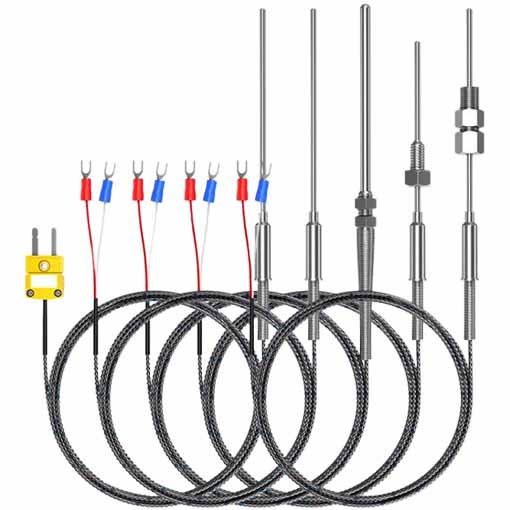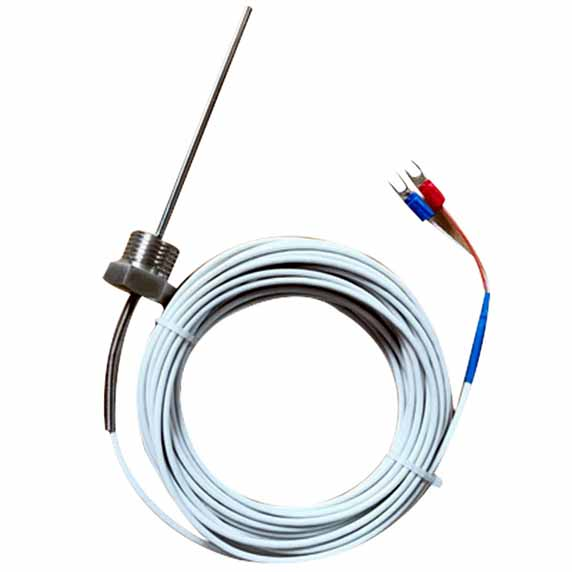China sensor manufacturers

# China wholesale thermocouple supplier

Product Item: China wholesale thermocouple supplier
Category: PT100 Sensor
The original factory-manufactured thermocouple is used for the following temperature detection: thermometer, water heater, fireplace, furnace, circuit, rtd, cold junction, oven, multimeter, digital, industrial

• PRODUCT DETAIL
The original factory-manufactured thermocouple is used for the following temperature detection: thermometer, water heater, fireplace, furnace, circuit, rtd, cold junction, oven, multimeter, digital, industrial

The working principle of thermocouple
When there are two different conductors or semiconductors A and B forming a loop, the two ends of which are connected to each other, as long as the temperatures at the two junctions are different. The temperature at one end is T, called the working end or hot end, and the temperature at the other end is T0, called the free end (also called the reference end) or cold end. An electromotive force will be generated in the loop. The direction and magnitude of the electromotive force are related to the material of the conductor and the temperature of the two junctions. This phenomenon is called "thermoelectric effect", and the loop composed of two conductors is called "thermocouple". These two conductors are called "thermoelectrodes", and the electromotive force generated is called "thermoelectromotive force".
The thermoelectromotive force consists of two parts of electromotive force, one part is the contact electromotive force of two conductors, and the other part is the thermoelectromotive force of a single conductor.
The size of the thermoelectromotive force in the thermocouple loop is only related to the temperature of the conductor material and the two junctions that make up the thermocouple, and has nothing to do with the shape and size of the thermocouple. When the two electrode materials of the thermocouple are fixed, the thermoelectromotive force is the difference of the function of the two junction temperature t and t0.

which is:
This relationship has been widely used in actual temperature measurement. Because the cold junction t0 is constant, the thermoelectromotive force generated by the thermocouple only changes with the temperature of the hot junction (measurement end), that is, a certain thermoelectromotive force corresponds to a certain temperature. We can achieve the purpose of temperature measurement as long as we use the method of measuring thermoelectromotive force.

The basic principle of thermocouple temperature measurement is that two conductors of different components form a closed loop. When there is a temperature gradient at both ends, there will be current passing through the loop. At this time, there is an electromotive force-thermoelectromotive force between the two ends, which is the so-called Seebeck effect. Two homogeneous conductors with different components are thermoelectrodes, the end with a higher temperature is the working end, the end with a lower temperature is the free end, and the free end is usually at a certain constant temperature. According to the functional relationship between thermoelectromotive force and temperature, a thermocouple index table is made;
The index table is obtained when the free end temperature is at 0℃, and different thermocouples have different index tables.

When the third metal material is connected in the thermocouple circuit, as long as the temperature of the two junctions of the material is the same, the thermoelectric potential generated by the thermocouple will remain unchanged. That is, it is not affected by the access of the third metal in the loop. Therefore, when the thermocouple measures the temperature, the measuring instrument can be connected, and the temperature of the measured medium can be known after the thermoelectromotive force is measured. When the thermocouple measures the temperature, the temperature of its cold junction (the measuring end is the hot end, and the end connected to the measurement circuit through the lead is called the cold junction) is required to maintain the same temperature. Its thermoelectric potential is proportional to the measured temperature. If the (environment) temperature of the cold junction changes during measurement, it will seriously affect the accuracy of the measurement. Take certain measures to compensate at the cold junction, and the influence caused by the temperature change of the cold junction is called the cold junction compensation of the thermocouple is normal. Dedicated compensation wire for connection with measuring instrument.

Calculation method of thermocouple cold junction compensation:
From millivolt to temperature: Measure the cold junction temperature, convert it to the corresponding millivolt value, add it to the millivolt value of the thermocouple, and calculate the temperature;
From temperature to millivolt: Measure the actual temperature of the cold end temperature, respectively, in terms of millivolts, after subtracting the values derived mV, to obtain the temperature.﻿
﻿